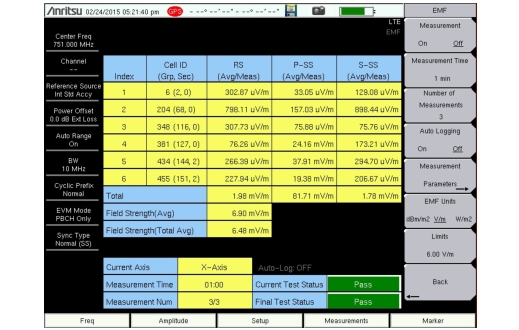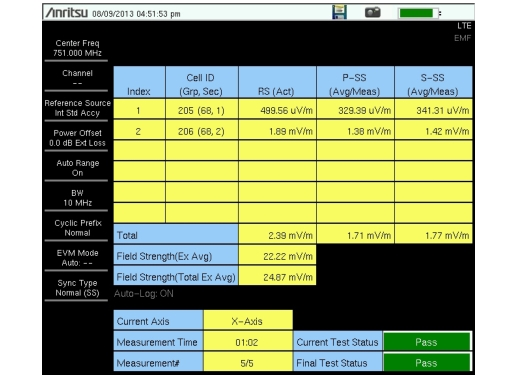Electromagnetic Field (EMF) MeasurementsOption 444 : LTE/TD-LTE OTA : Measurement Results (LTE/TD-LTE)

Measurement Results (LTE/TD-LTE)
The measurement starts by setting the antenna’s X axis and capturing over-the-air data. If a sync signal is found and a valid Cell ID exists, then the following parameters are detected and stored: the channel power in 1.4 MHz bandwidth, the Cell ID, RS, P-SS, and S-SS (all per Resource Element). This is repeated for Y and Z axes. If any one of the axes has a valid Cell ID, the isotropic result (for example, (RS2X + RS2Y + RS2Z)0.5) for each of the above parameters is displayed as the Actual result.
The Measurement Parameters submenu (refer to page Meas Params Menu (LTE/TD-LTE)) lets you choose which computed result is displayed in the measurements table, in each of the RS, P‑SS, and S‑SS columns. See Figure: LTE EMF Measurement Results. The choices of display parameters are: Actual, Total Min, Total Max, Avg/Meas (the default), and Total Avg.
Total Min, Total Max, and Total Avg are the min, max, and average values computed from all measurements completed thus far within the measurement period (Measurement Time × Number of Measurements). Avg/Meas is the running average of the isotropic results computed from all measurements completed thus far within the specified Measurement Time.There is no axis dwell time parameter. If a sync signal is not found within a specific time, data for the current axis is excluded and the instrument switches to the next axis. The Field Strength number is still computed and compared with the specified limit to determine the Pass or Fail status at the end of the measurement period (Measurement Time × Number of Measurements).
Field Strength (Avg) is the running average for the current Measurement Time. Field Strength (Total Avg) is the running average for all measurements completed thus far within the measurement period.
When the extrapolation factor is turned Off, the Field Strength number is the measured Channel Power in a 1.4 MHz bandwidth. Changing the bandwidth (BW) setting in the Setup menu does not change this number.
If the extrapolation factor is On, the Field Strength (Emax) is computed as follows:
Emax = Ecp × Ncp
where Ecp is the RMS value of the channel power recorded in each axis and Ncp is the number of subcarriers divided by 72. The number of subcarriers can be provided by the network operator or can be calculated from Table: Field Strength Numbers. The selected channel bandwidth (BW key in the instrument Setup main menu) determines the number of subcarriers. The default BW is 1.4 MHz.
 Channel Bandwidth (MHz) Subcarriers 1.4 72 3 180 5 300 10 600 15 900 20 1200
Assuming that all subcarriers in the BW setup are at the same power level, the Field Strength value for other BW setups can be extrapolated based on the Channel Power in 1.4 MHz BW. The Field Strength cell labels in the table are updated with an Ex, such as Field Strength (Ex Avg), to indicate the extrapolation factor has been applied. See Figure: LTE/TD-LTE EMF Measurement Display.
The displayed values are measurement results from the BW setup made prior to starting the measurement. Changing the BW setup, hence the extrapolation factor, after the measurement is complete has no effect on the currently displayed values.
If a valid Cell ID is obtained even once during the entire measurement period, an entry will be made in the table. “--” indicates an invalid result. A maximum of six cell IDs can be detected. The Total row sums the isotropic numbers for the selected display parameter across Cell IDs.
Pass/Fail
The limit check is done at the end of each Measurement Time. If the Field Strength (Avg), with or without extrapolation, exceeds the set limit, the Current and the Final Test Status are marked as Fail in red. If the Field Strength (Avg) does not exceed the limit, the Current Test Status is marked as Pass in green. In the example in Figure: LTE/TD-LTE EMF Measurement Display, the extrapolated Field Strength (Ex Avg) is 22.22 mV/m.
If all of the measurements pass, the Final Test Status is updated to Pass in green.